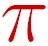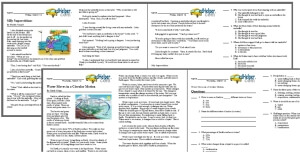Worksheets and No Prep Teaching Resources Reading Comprehension Worksheets March Worksheets Pi DayPi Day

History of Pi

Print History of Pi Reading Comprehension

Vocabulary
 challenging words: irrational, polygon, circumference, calculus, estimation, calculation, finding, geometry, radius, mathematician, based, formula, tombstone, area, decimal, ratio content words: Ludolph Van Ceulen, Johann Lambert, Srinivasa Ramanujan
History of Pi

By Sharon Fabian
1     To find the area of a circle, you multiply pi times the radius of the circle squared. To find the circumference of a circle, you multiply pi times the circle's diameter. Pi is a useful number, but before we could use it, someone had to figure out that there was such a thing as pi.

2     Pi is the ratio of the circumference to the diameter of a circle. It is the same number for every circle. The digits for the number pi begin 3.1415926535 and continue without ever ending or repeating. We often use a shorter estimation for pi, such as 3.14.

3     In ancient times, people in China, Babylon, and Egypt used pi. Even the Bible mentions circle calculations that are based on the number pi. In those times people were not using decimals yet, so they used either the whole number 3 or fractions for pi.

Paragraphs 4 to 10:

English Reading Comprehension: History of Pi
French Reading Comprehension: L'Histoire de Pi
Spanish Reading Comprehension: La Historia de Pi
Italian Reading Comprehension: Storia del pi greco
German Reading Comprehension: Die Geschichte der Zahl Pi

History of Pi Math Printable
History of Pi Math Problems

Feedback on History of PiCreate Weekly Reading Books Prepare for an entire week at once!